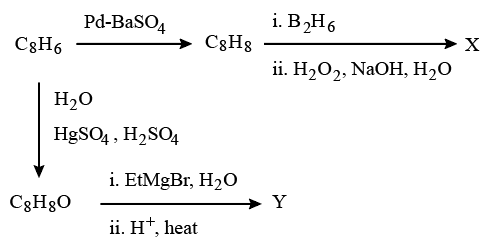Instructions

For the following questions answer them individually

Question 31

Question 32

Question 33

Question 34

Question 35

Question 36

# One mole of a monoatomic real gas satisfies the equation $$p(V - b) = RT$$ where b is a constant. The relationship of interatomic potential V(r) and interatomic distance r for the gas is given by

Instructions

In the following reactionsQuestion 37

Question 38

# The major compound Y is

Instructions

When 100 mL of 1.0 M HCl was mixed with 100 mL of 1.0 M NaOH in an insulated beaker at constant pressure, a temperature increase of $$5.7 ^{\circ}C$$ was measured for the beaker and its contents (Expt. 1). Because the enthalpy of neutralization of a strong acid with a strong base is a constant $$(-57.0 kJ mol^{-1})$$, this experiment could be used to measure the calorimeter constant. In a second experiment (Expt. 2), 100 mL of 2.0 M acetic acid $$(K_a = 2.0 \times 10^{-5})$$ was mixed with 100 mL of 1.0 M NaOH (under identical conditions to Expt. 1) where a temperaturerise of $$5.6 ^{\circ}C$$ was measured.
(Consider heat capacity of all solutions as $$4.2 J g^{-1} K^{-1}$$ and densityof all solutions as $$1.0 g mL^{-1})$$

Question 39

Question 40

OR